# 时间序列异常检测算法综述

## 异常的分类

• 革新性异常：innovational outlier (IO)，造成离群点的干扰不仅作用于$X_T$，而且影响T时刻以后序列的所有观察值。
• 水平移位异常：level shift (LS)，造成这种离群点的干扰是在某一时刻T，系统的结构发生了变化，并持续影响T时刻以后的所有行为，在数列上往往表现出T时刻前后的序列均值发生水平位移。
• 暂时变更异常temporary change (TC)：造成这种离群点的干扰是在T时刻干扰发生时具有一定初始效应，以后随时间根据衰减因子的大小呈指数衰减。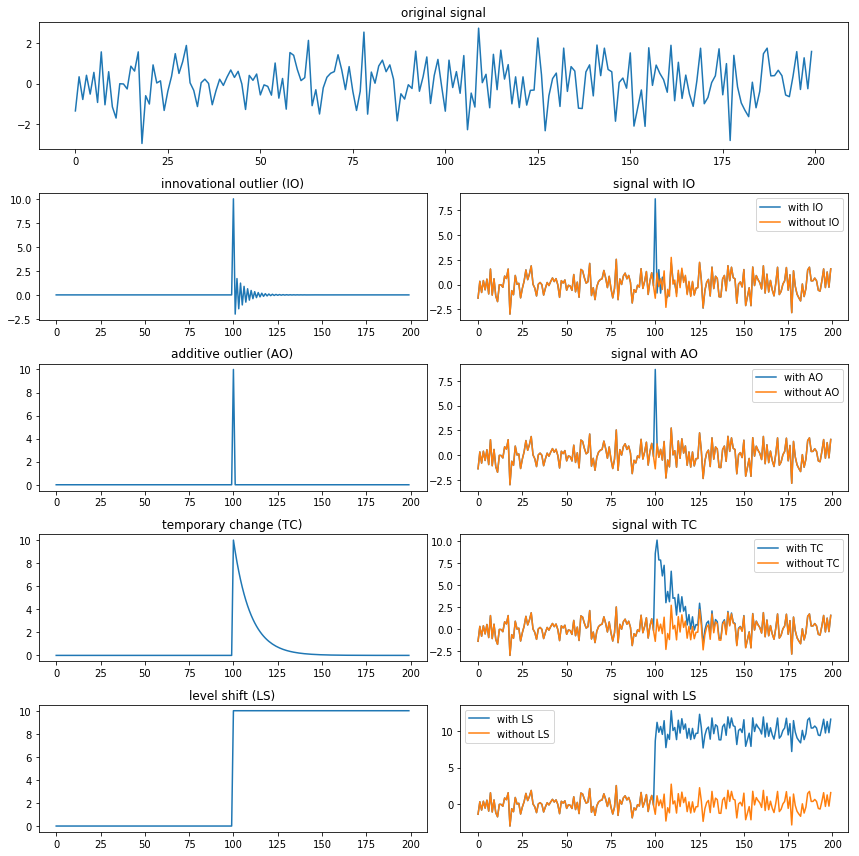## 常见异常检测方法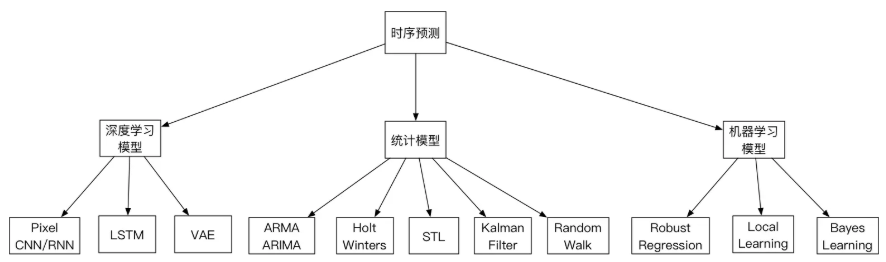• 统计模型：优点是复杂度低，计算速度快，泛化能力强悍。因为没有训练过程，即使没有前期的数据积累，也可以快速的投入生产使用。缺点是准确率一般。但是这个其实是看场景的，并且也有简单的方法来提高业务层面的准确率。这个后面会提到。
• 机器学习模型：鲁棒性较好，准确率较高。需要训练模型，泛化能力一般。
• 深度学习模型：普遍需要喂大量的数据，计算复杂度高。整体看，准确性高，尤其是近段时间，强化学习的引入，进一步巩固其准确性方面的领先优势。

### 3-Sigma

3-Sigma原则又称为拉依达准则，该准则定义如下：假设一组检测数据只含有随机误差，对原始数据进行计算处理得到标准差，然后按一定的概率确定一个区间，认为误差超过这个区间的就属于异常值。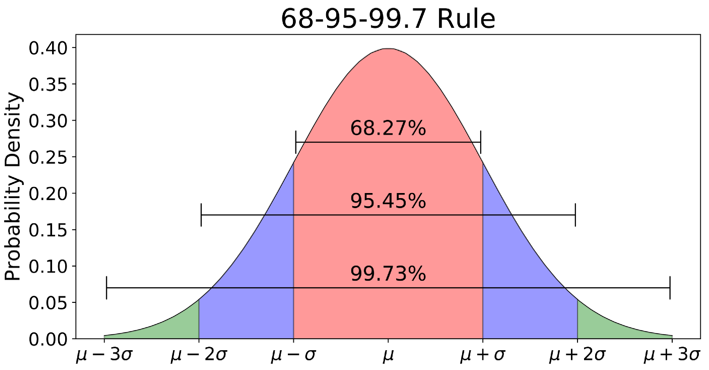import numpy as np

def three_sigma(df_col):
'''
df_col：DataFrame数据的某一列
'''
rule = (df_col.mean() - 3 * df_col.std() > df_col) | (df_col.mean() + 3 * df_col.std() < df_col)
index = np.arange(df_col.shape)[rule]
out_range = df_col.iloc[index]
return out_range


• 删除带有异常值的数据；
• 将异常值视为缺失值，交给缺失值处理方法来处理；
• 用平均值进行修正；
• 当然我们也可以选择不处理。

### Grubbs测试

Grubbs’Test为一种假设检验的方法，常被用来检验服从正太分布的单变量数据集（univariate data set）Y 中的单个异常值。若有异常值，则其必为数据集中的最大值或最小值。原假设与备择假设如下：

• $H_0$: 数据集中没有异常值
• $H_1$: 数据集中有一个异常值

Grubbs’ Test检验假设的所用到的检验统计量（test statistic）为：

$$G = \frac{\max |Y_i – \overline{Y}|}{s}$$

$$G > \frac{(N-1)}{\sqrt{N}}\sqrt{\frac{ (t_{\alpha/(2N), N-2})^2}{N-2 + (t_{\alpha/(2N), N-2})^2}}$$

1. 样本从小到大排序
2. 求样本的mean和dev
3. 计算min/max与mean的差距，更大的那个为可疑值
4. 求可疑值的z-score (standard score)，如果大于Grubbs临界值，那么就是outlier

Grubbs临界值可以查表得到，它由两个值决定：检出水平$\alpha$（越严格越小），样本数量n，排除outlier，对剩余序列循环做 1-4 步骤。由于这里需要的是异常判定，只需要判断tail_avg是否outlier即可。

from outliers import smirnov_grubbs as grubbs

print(grubbs.test([8, 9, 10, 1, 9], alpha=0.05))
print(grubbs.min_test_outliers([8, 9, 10, 1, 9], alpha=0.05))
print(grubbs.max_test_outliers([8, 9, 10, 1, 9], alpha=0.05))
print(grubbs.max_test_indices([8, 9, 10, 50, 9], alpha=0.05))

### ESD（generalized ESD test）

1. 计算与均值偏离最远的残差，注意计算均值时的数据序列应是删除上一轮最大残差样本数据后。$R_j = \frac{\max_i |Y_i – \overline{Y’}|}{s}, 1 \leq j \leq k$
2. 计算临界值（critical value）.$\lambda_j = \frac{(n-j) * t_{p,n-j-1}}{\sqrt{(n-j-1+t_{p,n-j-1}^2)(n-j+1)}}, 1 \leq j \leq k$
3. 检验原假设，比较检验统计量与临界值；若$R_i > \lambda_j$，则原假设$H_0$不成立，该样本点为异常点
4. 重复以上步骤k次至算法结束。

import numpy as np
import matplotlib.pylab as plt
from PyAstronomy import pyasl

# Convert data given at:
# http://www.itl.nist.gov/div898/handbook/eda/section3/eda35h3.htm
# to array.
x = np.array([float(x) for x in "-0.25 0.68 0.94 1.15 1.20 1.26 1.26 1.34 1.38 1.43 1.49 1.49 \
1.55 1.56 1.58 1.65 1.69 1.70 1.76 1.77 1.81 1.91 1.94 1.96 \
1.99 2.06 2.09 2.10 2.14 2.15 2.23 2.24 2.26 2.35 2.37 2.40 \
2.47 2.54 2.62 2.64 2.90 2.92 2.92 2.93 3.21 3.26 3.30 3.59 \
3.68 4.30 4.64 5.34 5.42 6.01".split()])

# Apply the generalized ESD
r = pyasl.generalizedESD(x, 10, 0.05, fullOutput=True)

print("Number of outliers: ", r)
print("Indices of outliers: ", r)
print("        R      Lambda")
for i in range(len(r)):
print("%2d  %8.5f  %8.5f" % ((i + 1), r[i], r[i]))

# Plot the "data"
plt.plot(x, 'b.')
# and mark the outliers.
for i in range(r):
plt.plot(r[i], x[r[i]], 'rp')
plt.show()


### S-ESD与S-H-ESD

STL分解

STL (Seasonal-Trend decomposition procedure based on Loess) 为时序分解中一种常见的算法，基于LOESS将某时刻的数据$Y_v$分解为趋势分量（trend component）、季节性分量（seasonal component）和残差（remainder component）:

$$Y_v = T _v + S_v + R_v \quad v= 1, \cdots, N$$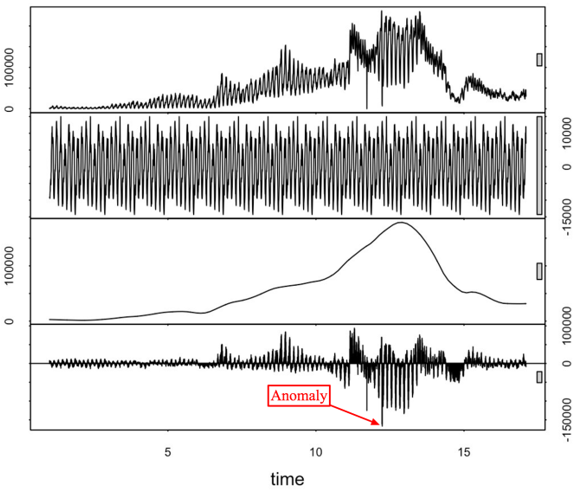STL分为内循环（inner loop）与外循环（outer loop），其中内循环主要做了趋势拟合与周期分量的计算。假定$T_v^{(k)}$、Sv(k)为内循环中第k-1次pass结束时的趋势分量、周期分量，初始时T(k)v=0；并有以下参数：

• n(i)内层循环数
• n(o)外层循环数
• n(p)为一个周期的样本数
• n(s)为Step 2中LOESS平滑参数
• n(l)为Step 3中LOESS平滑参数
• n(t)为Step 6中LOESS平滑参数

• Step 1: 去趋势（Detrending），减去上一轮结果的趋势分量，$Y_v – T_v^{(k)}$
• Step 2: 周期子序列平滑（Cycle-subseries smoothing），用$\text{LOESS}(q=n_{n(s)}, d=1)$对每个子序列做回归，并向前向后各延展一个周期；平滑结果组成temporary seasonal series，记为$C_v^{(k+1)}, v = -n_{(p)} + 1, \cdots, -N + n_{(p)}$
• Step 3: 周期子序列的低通量过滤（Low-Pass Filtering），对上一个步骤的结果序列$C_v^{(k+1)}$依次做长度为n(p)、n(p)、3的滑动平均（moving average），然后做$\text{LOESS}(q=n_{n(l)}, d=1)$回归，得到结果序列$L_v^{(k+1)}, v = 1, \cdots, N$；相当于提取周期子序列的低通量
• Step 4: 去除平滑周期子序列趋势（Detrending of Smoothed Cycle-subseries），$S_v^{(k+1)} = C_v^{(k+1)} – L_v^{(k+1)}$
• Step 5: 去周期（Deseasonalizing），减去周期分量，$Y_v – S_v^{(k+1)}$
• Step 6: 趋势平滑（Trend Smoothing），对于去除周期之后的序列做$\text{LOESS}(q=n_{n(t)}, d=1)$回归，得到趋势分量$T_v^{(k+1)}$。

$$h = 6 * median(|R_v|)$$

$$\rho_v = B(|R_v|/h)$$

$$B(u) = \left\{\begin{matrix}(1-u^2)^2 & 0 \le u < 1 \\ 0 & u \ge 1 \end{matrix}\right.$$

outer loop:
计算robustness weight;
inner loop:
Step 1 去趋势;
Step 2 周期子序列平滑;
Step 3 周期子序列的低通量过滤;
Step 4 去除平滑周期子序列趋势;
Step 5 去周期;
Step 6 趋势平滑;


Python的statsmodels实现了一个简单版的时序分解，通过加权滑动平均提取趋势分量，然后对cycle-subseries每个时间点数据求平均组成周期分量：

import numpy as np
import pandas as pd
from statsmodels.tsa.seasonal import seasonal_decompose
import matplotlib.pyplot as plt

# Generate some data
np.random.seed(0)
n = 1500
dates = np.array('2019-01-01', dtype=np.datetime64) + np.arange(n)
data = 12 * np.sin(2 * np.pi * np.arange(n) / 365) + np.random.normal(12, 2, 1500)
df = pd.DataFrame({'data': data}, index=dates)

# Reproduce the example in OP
plt.show()


S-ESD

STL将时间序列数据分解为趋势分量、周期分量和余项分量。想当然的解法——将ESD运用于STL分解后的余项分量中，即可得到时间序列上的异常点。但是，我们会发现在余项分量中存在着部分假异常点（spurious anomalies）。如下图所示：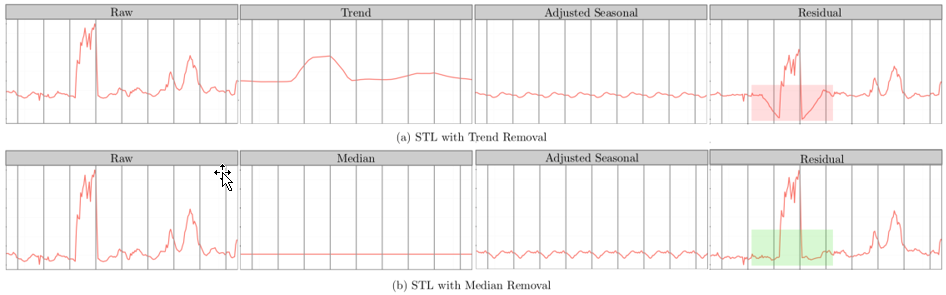$$R_X = X – S_X- \tilde{X}$$

import numpy as np
import sesd
ts = np.random.random(100)
# Introduce artificial anomalies
ts = 9
ts = 10
outliers_indices = sesd.seasonal_esd(ts, periodicity=20, hybrid=True, max_anomalies=2)
for idx in outliers_indices:
print(f'Anomaly index: {idx}, anomaly value: {ts[idx]}')


S-H-ESD

$$\text{MAD} = median(|X_i – median(X)|)$$

from pyculiarity import detect_ts
import matplotlib.pyplot as plt
import pandas as pd

# first run the models
results = detect_ts(twitter_example_data, max_anoms=0.05, alpha=0.001, direction='both', only_last=None)

# format the twitter data nicely

# make a nice plot
f, ax = plt.subplots(2, 1, sharex=True)
ax.plot(results['anoms'].index, results['anoms']['anoms'], 'ro')
ax.set_title('Detected Anomalies')
ax.set_xlabel('Time Stamp')
ax.set_ylabel('Count')
ax.plot(results['anoms'].index, results['anoms']['anoms'], 'b')
ax.set_ylabel('Anomaly Magnitude')
plt.show()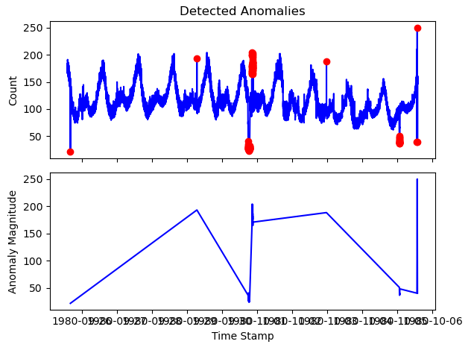PyCuliarity的使用详解

PyCuliarity有两个顶级函数，一个用于timeseries数据，一个用于向量数据处理，detect_ts和 detect_vec：

• detect_ts：输入的DataFrame需要两列数据，其中一列为时间，另一列为该时间点对应的值
• detect_vec：可以不包含是时间列，时间索引按照DataFrame长度自动生成。

def detect_ts(df, max_anoms=0.10, direction='pos',
alpha=0.05, only_last=None, threshold=None,
e_value=False, longterm=False,
piecewise_median_period_weeks=2, plot=False,
y_log=False, xlabel = '', ylabel = 'count',
title=None, verbose=False):
"""
Anomaly Detection Using Seasonal Hybrid ESD Test
A technique for detecting anomalies in seasonal univariate time series where the input is a
series of <timestamp, value> pairs.

Args:

x: Time series as a two column data frame where the first column consists of the
timestamps and the second column consists of the observations.

max_anoms: Maximum number of anomalies that S-H-ESD will detect as a percentage of the
data.

direction: Directionality of the anomalies to be detected. Options are: ('pos' | 'neg' | 'both').

alpha: The level of statistical significance with which to accept or reject anomalies.

only_last: Find and report anomalies only within the last day or hr in the time series. Options: (None | 'day' | 'hr')

threshold: Only report positive going anoms above the threshold specified. Options are: (None | 'med_max' | 'p95' | 'p99')

e_value: Add an additional column to the anoms output containing the expected value.

longterm: Increase anom detection efficacy for time series that are greater than a month.

See Details below.
piecewise_median_period_weeks: The piecewise median time window as described in Vallis, Hochenbaum, and Kejariwal (2014). Defaults to 2.

plot: (Currently unsupported) A flag indicating if a plot with both the time series and the estimated anoms,
indicated by circles, should also be returned.

y_log: Apply log scaling to the y-axis. This helps with viewing plots that have extremely
large positive anomalies relative to the rest of the data.

xlabel: X-axis label to be added to the output plot.
ylabel: Y-axis label to be added to the output plot.

Details

'longterm' This option should be set when the input time series is longer than a month.
The option enables the approach described in Vallis, Hochenbaum, and Kejariwal (2014).
'threshold' Filter all negative anomalies and those anomalies whose magnitude is smaller
than one of the specified thresholds which include: the median
of the daily max values (med_max), the 95th percentile of the daily max values (p95), and the
99th percentile of the daily max values (p99).
'title' Title for the output plot.
'verbose' Enable debug messages

The returned value is a dictionary with the following components:
anoms: Data frame containing timestamps, values, and optionally expected values.
plot: A graphical object if plotting was requested by the user. The plot contains
the estimated anomalies annotated on the input time series
"""


• df：包含时间和值的DataFrame
• max_anoms=0.10：发现异常数据的量（占总体的百分之多少）
• direction=’pos’：’pos’是发现数据突增点，’neg’是发现数据突降点，’both’是包含突增与突降
• alpha=0.05：接受或拒绝显著性水平，即p-value
• only_last=None：仅再时间序列最后1天（’day’）或1小时（’hr’）寻找异常
• threshold=None：仅报告高于指定阈值的正向异常。选项有：
• med_max：每日最大值的中位数
• p95：每日最大值的95%
• p99：每日最大值的99%
• e_value=False：返回数据中新增一列期望值（加了后都是NaN，不清楚原因）
• longterm=False：当时间序列超过一个月时，设置此值，
• piecewise_median_period_weeks=2：当设置longterm后需要设置该值，设置滑动窗口的大小，注意这里需要>=2
• plot=False：输出图像，已经不支持
• y_log=False：对Y轴值取对数
• xlabel = ”：添加输出到图形的X轴标签
• ylabel = ‘count’：添加输出到图形的Y轴标签
• title=None：输出图像的标签
• verbose=False：是否输出debug信息

### 移动平均/加权移动平均/指数加权移动平均

\begin{align*} CMA_{i+1} &= \frac{x_1+…+x_i+x_{i+1}}{i+1} \\ & = \frac{x_{i+1}+n·CMA_i}{i+1}\\ & = CMA_i + \frac{x_{i+1}-CMA_i}{i+1} \end{align*}

• 并没有时间窗口，用的是从时间序列第一个data point到当前data point之间的所有点。
• 每个data point的权重不同，离当前时间点越近的点的权重越大，历史时间点的权重随着离当前时间点的距离呈指数衰减，从当前data point往前的data point，权重依次为$\alpha ,\alpha (1-\alpha),\alpha (1-\alpha)^2,…,\alpha (1-\alpha)^n$。

$$S_t = \alpha Y_t+(1-\alpha)(S_{t-1}+b_{t-1})$$

$$b_t = \beta(S_t-S_{t-1})+(1-\beta)b_{t-1}$$

$$b_1 = Y_2-Y_1$$

$$b_1 = \frac{(Y_2-Y_1)+(Y_3-Y_2)+(Y_4-Y_3)}{3}=\frac{Y_4-Y_1}{3}$$

$$b_1 = \frac{Y_n-Y_1}{n-1}$$

• 单个时刻：$F_{t+1}=S_t+b_t$
• m个时刻：$F_{t+m}=S_t+mb_t$

multiplicative seasonality

$$S_t = \alpha \frac{Y_t}{c_{t-L}}+(1-\alpha)(S_{t-1}+b_{t-1}),overall\ smoothing$$

$$b_t = \beta(S_t-S_{t-1})+(1-\beta)b_{t-1},trend\ smoothing$$

$$c_t = \gamma\frac{Y_t}{S_t}+(1-\gamma)c_{t-L}, seasonal smoothing$$

$$S_1 = Y_1$$

$$b_0 = \frac{(Y_{L+1}-Y_1)+(Y_{L+2}-Y_2)+…+(Y_{L+L}-Y_L)}{L}$$

$$c_i = \frac{1}{N}\sum_{j=1}^N\frac{Y_{L(j-1)+i}}{A_j}, \forall i \in \{1,…,L\}$$

$$A_j=\frac{\sum_{i=1}^LY_{L(j-1)+i}}{L}, \forall \in \{1,…,N\}$$

$$S_t = \alpha(Y_t-c_{t-L})+(1-\alpha)(S_{t-1}+b_{t-1}), overall \ smoothing$$

$$b_t = \beta(S_t- S_{t-1})+(1-\beta)b_{t-1}, trend \ smoothing$$

$$c_t = \gamma(Y_t-S_{t-1}-b_{t-1})+(1-\gamma)c_{t-L}, seasonal smoothing$$

### ARIMA 模型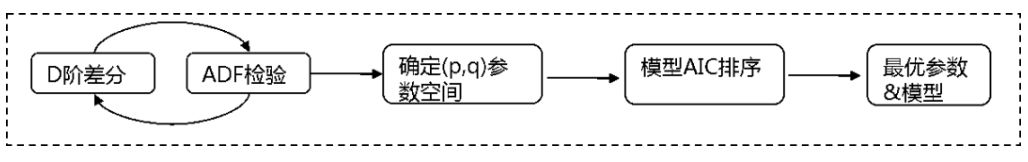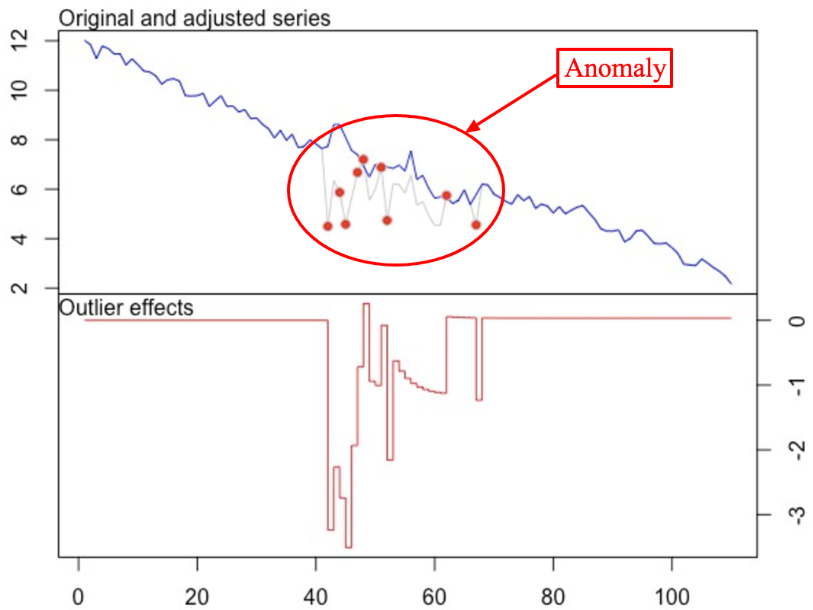## 时间序列异常检测方案

### 360时间序列检测机制

$$count(\sum_{i=0}^{T}(nowvalue-i)>threshold)>count\_num$$

Threshold可以采用动态阈值。业界关于动态阈值设置的方法有很多，通常阈值设置方法会参考过去一段时间内的均值、最大值以及最小值，我们也同样应用此方法。取过去一段时间（比如 T 窗口）的平均值、最大值以及最小值，然后取 max-avg 和 avg-min 的最小值。之所以取最小值的原因是让筛选条件设置的宽松一些，让更多的值通过此条件，减少一些漏报的事件。

$$threshold = min(max-avg,avg-min)$$

$$amplitude=max[\left | \frac{x_i(t)-x_i(t-1)}{x_i(t-1}\right |],x=1,2,…,14$$

1、根据周期性的不同来选择合适的方法。这种方法需要首先验证序列是否具有周期性，如果具有周期性则进入左边分支的检测方法，如果没有周期性则选择进入右分支的检测方法。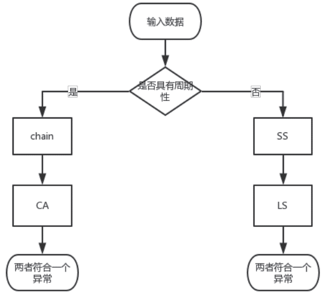2、不区分周期性，直接根据“少数服从多数”的方法来去检测，这种方法比较好理解，在此就不说明了。### 美团外卖订单量预测异常报警模型

• 异常驱动的异常检测（敏感性）：宁愿误报，也不能错过任何一个异常，这适用于非常重要的检测。简单概括，就是“宁可错杀一千，不能放过一个”。
• 预算驱动的异常检测（准确性）：这种策略的异常检测，从字面理解就是只有定量的一些预算去处理这些报警，那么只能当一定是某种问题时，才能将报警发送出来。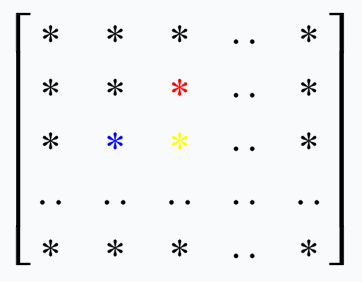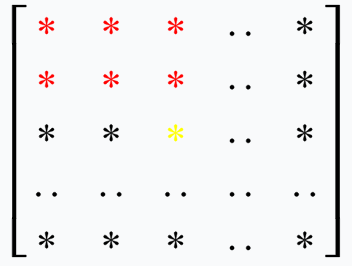Holt-Winters预测器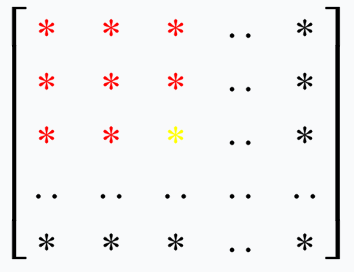1、使用Holt-Winters算法计算周期数据

• 优点：如果序列中含有周期性的陡增陡降点，Holt-Winters计算出的周期数据中会保留这些陡增陡降趋势，因此可以准确的预测出这些趋势，不会产生误报。比如外卖订单的提单数据，在每天的某个时刻都有一个定期陡降，使用该方式可以正确的预测出下降的趋势。如图所示，蓝色线是真实数据，棕色线是预测数据，在该时刻，棕色线准确的预测出了下降点。
• 缺点：需要对输入数据进行预处理，去除异常数据。如果输入序列中含有异常数据，使用Holt-Winters时可能会把这些异常数据计算到周期数据中，影响下一周期的预测从而产生误报（Holt-Winters理论上也只是滑动平均的过程，因此如果输入数据中含有比较大的异常数据时，存在这种可能性，实际应用中订单的报警模型也出现过这种误报）。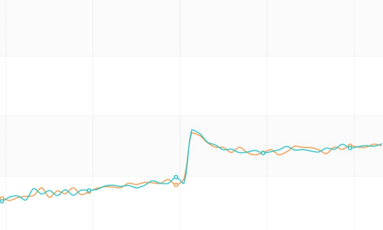2、历史数据平均求基线

• 优点：计算出的周期数据比较平滑，不需要对输入序列进行预处理，计算过程中可以自动屏蔽掉异常数据的影响，计算过程简单。
• 缺点：周期数据比较平滑，不会出现陡增陡降点，因此对于周期性出现的陡增陡降不能很好的预测，出现误报。比如外卖活动的大盘（如图所示，红线是真实数据，黑线是预测数据），提前下单优惠在每天某个时刻会出现周期性陡降，使用该方式会出现误报。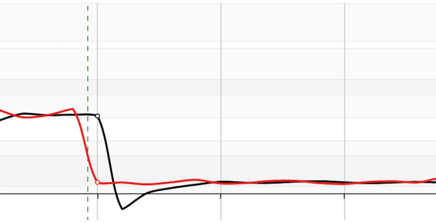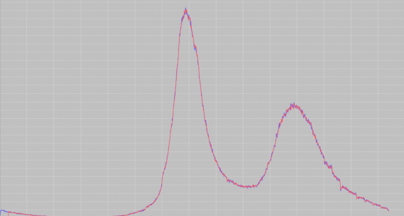• 离散度Filter：根据预测误差曲线离散程度过滤出可能的异常点。一个序列的方差表示该序列离散的程度，方差越大，表明该序列波动越大。如果一个预测误差序列方差比较大，那么我们认为预测误差的报警阈值相对大一些才比较合理。离散度Filter利用了这一特性，取连续15分钟的预测误差序列，分为首尾两个序列（e1,e2），如果两个序列的均值差大于e1序列方差的某个倍数，我们就认为该点可能是异常点。
• 阈值Filter：根据误差绝对值是否超过某个阈值过滤出可能的异常点。利用离散度Filter进行过滤时，报警阈值随着误差序列波动程度变大而变大，但是在输入数据比较小时，误差序列方差比较小，报警阈值也很小，容易出现误报。所以设计了根据误差绝对值进行过滤的阈值Filter。阈值Filter设计了一个分段阈值函数y=f(x)，对于实际值x和预测值p，只有当|x-p|>f(x)时报警。实际使用中，可以寻找一个对数函数替换分段阈值函数，更易于参数调优。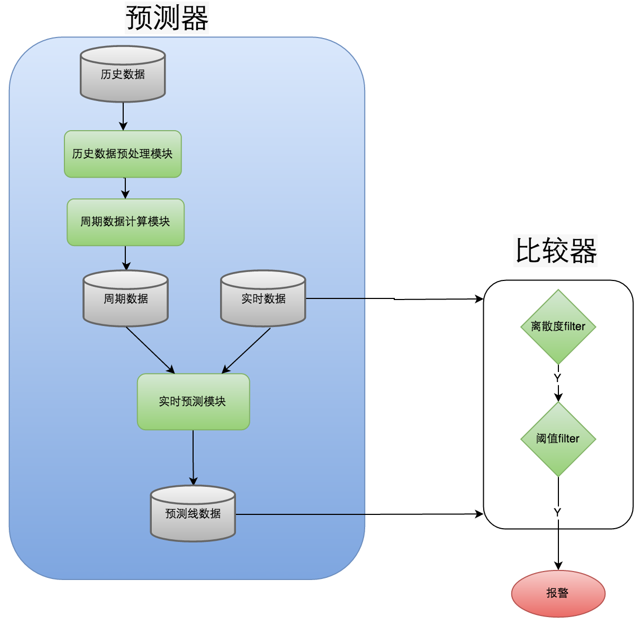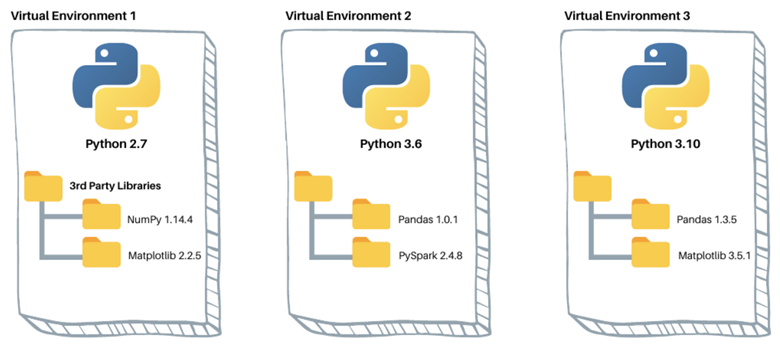##### Python虚拟环境的创建与管理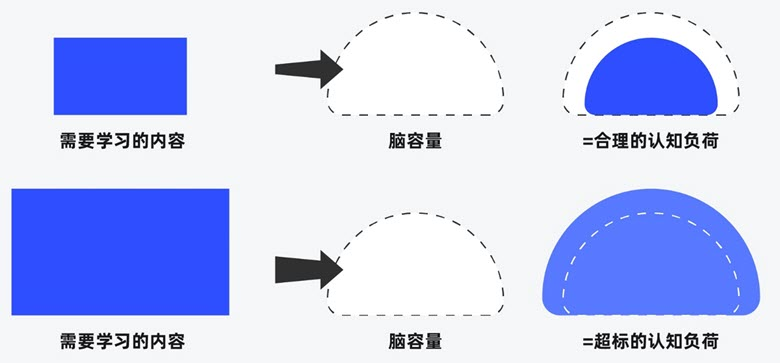##### 界面设计和游戏设计中的认知负荷